# Context-sensitive L-systems

(back to contents)

In the second chapter it was said that L-systems are a variant of formal grammars. Like regular context-sensitive grammars, context-sensitive L-systems try to match text on both sides of a symbol before doing a rewrite. The difference is that matching is more elaborate compared to regular context-sensitive grammars. To quote "The Algorithmic Beauty of Plants" book (text refers to brackets in the sense of square brackets - turtle stack commands):

The introduction of context to bracketed L-systems is more difficult than in L-systems without brackets, because the bracketed string representation of axial trees does not preserve segment neighborhood. Consequently, the context matching procedure may need to skip over symbols representing branches or branch portions.
To illustrate differences let's consider an example. One of the uses of a context-sensitive L-system is signal propagation. If symbol `S` represents a signal (for example flower-inducing hormone) and symbol `B` represents a piece of stem, then signal propogation along such a stem could be modeled by the following L-system:
```(with-debug
(iteration-count 9)
(set-axiom (S B B B B B B B B))
(add-rule ((S < B     -> S)
(    B     -> B)))
(add-rule ((    S > B -> B)
(    S     -> S))))
```
If `B` has `S` on the left side, then become `S`, else stay as `B`.
If `S` has `B` on the right side, then become `B`, else stay as `S`.
This is how it rewrites:
 axiom: `(S B B B B B B B B)` p1: `(B S B B B B B B B)` p2: `(B B S B B B B B B)` p3: `(B B B S B B B B B)` p4: `(B B B B S B B B B)` p5: `(B B B B B S B B B)` p6: `(B B B B B B S B B)` p7: `(B B B B B B B S B)` p8: `(B B B B B B B B S)` p9: `(B B B B B B B B S)`
Now assume that the stem has branches. In that case the signal should be propagated in all sub branches and in the main trunk as well. The thing is that a sub branch must consider its parent branch as the part of its left context, while the main brach must ignore sub branches in its left context. Therefore L-system left context is matched differenty than that in regular grammars. For example in the word `(A B [ C D [ X Y ] E [ O ])`, symbol `O` will consider `(A B C D E)` as its left context. Notice that in the given example symbols `+` and `-` are ignored. In fact all predefined rules except `[` and `]` are ignored. To get a context matcher to ignore user defined symbols, its rule must start with the `:nameless` keyword. For example in the word `(S B B B C B B B B B)`, symbol `C` would block signal `S` from propagating unless its rule is specified in the following way `(:nameless C -> C)`.
 axiom: `(S B B B [ - B B B B B ] [ + B B B B B ] B B B B B)` p1: `(B S B B [ - B B B B B ] [ + B B B B B ] B B B B B)` p2: `(B B S B [ - B B B B B ] [ + B B B B B ] B B B B B)` p3: `(B B B S [ - B B B B B ] [ + B B B B B ] B B B B B)` p4: `(B B B B [ - S B B B B ] [ + S B B B B ] S B B B B)` p5: `(B B B B [ - B S B B B ] [ + B S B B B ] B S B B B)` p6: `(B B B B [ - B B S B B ] [ + B B S B B ] B B S B B)` p7: `(B B B B [ - B B B S B ] [ + B B B S B ] B B B S B)` p8: `(B B B B [ - B B B B S ] [ + B B B B S ] B B B B S)` p9: `(B B B B [ - B B B B S ] [ + B B B B S ] B B B B S)`
Graphically it looks something like this:
 axiom p1 p2 p3 p4 p5 p6 p7 p8 p9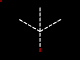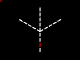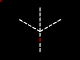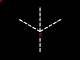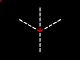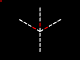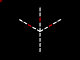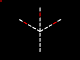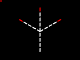On the other hand, the right context is matched similarly like in regular grammars. For example the rule `(A > [ B ] F -> ...)` does match `A` in `(A [ B ] F G)`, but does not match `A` in `(A [ B C ] F G)`. However, there is a small feature to skip branches in the right context. It is a special symbol `*` that matches anything until the corresponding closing square bracket. For example `(A > [ B * ] F -> ...)` now matches `A` in `(A [ B C [ D ] E ] F G)`.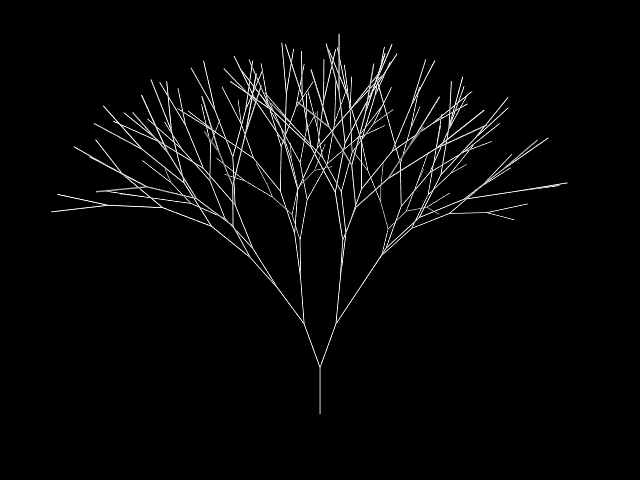```(defconstant +fibonacci+ 137.50777) (defun segment () (turtle-forward 80.0)) (with-graphics (iteration-count 7) (add-macro (R -> roll((- +fibonacci+)))) (set-axiom (R B(:active))) (add-option B (segment)) (add-rule ((B(x) ? (eq x :active) -> B(:inactive) roll(+fibonacci+) [ +(20) B(:active) ] [ -(20) B(:active) ]) (B(x) -> B(x))))) ```

First example describes simple branching structure where each branch splits into two sub branches.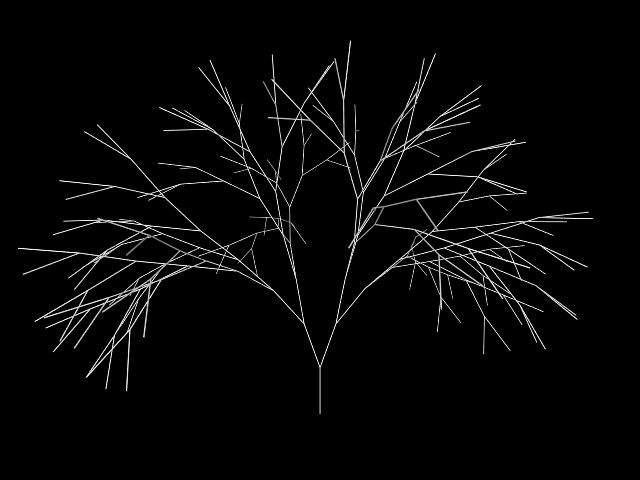```(defconstant +fibonacci+ 137.50777) (defparameter *gravity* (create-point 0.0 -1.0 0.0)) (defun segment () (turtle-tropism 80.0 *gravity* 0.3)) (with-graphics (iteration-count 7) (add-macro (R -> roll((- +fibonacci+)))) (set-axiom (R B(:active))) (add-option B (segment)) (add-rule ((B(x) ? (eq x :active) -> B(:inactive) roll(+fibonacci+) [ +(20) B(:active) ] [ -(20) B(:active) ]) (B(x) -> B(x))))) ```

Second example introduces new turtle manipulation function `turtle-tropism`. Tropism function takes three arguments: distance to go forward, tropism vector and tropism strength. Tropism function is similar to `forward` command in a way that it moves turtle forward, except that `turtle-tropism` biases turtle movement in a direction specified by tropism vector multiplied by tropism strength. In this particular example tropism vector points down hence the name `*gravity*`, but it can point sideways to simulate wind tropism or upwards to simulate sun tropism.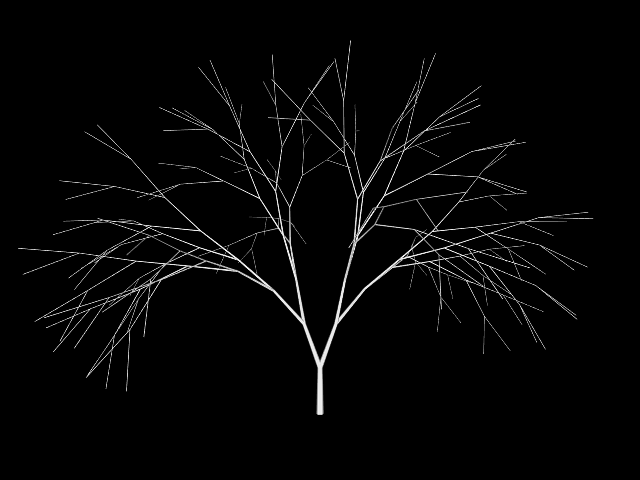```(defconstant +fibonacci+ 137.50777) (defparameter *gravity* (create-point 0.0 -1.0 0.0)) (defun segment (s) (turtle-set-surface s) (turtle-tropism 80.0 *gravity* 0.3)) (with-graphics (iteration-count 14) (add-macro (R -> roll((- +fibonacci+)))) (set-axiom (R B(:active 1.0))) (add-option B (segment s)) (add-rule ((B(x s) > [ B(x1 s1) * ] [ B(x2 s2) * ] ? (<= 7 *iterations-so-far*) -> B(x (+ s1 s2))) (B(x s) ? (<= 7 *iterations-so-far*) -> B(x s)) (B(x s) ? (eq x :active) -> B(:inactive s) roll(+fibonacci+) [ +(20) B(:active 1.0) ] [ -(20) B(:active 1.0) ]) (B(x s) -> B(x s))))) ```

Third example introduces context-sensitive rule. Context-sensitive rule is used to associate weigth with branches in such way that each "leaf" branch has the weight of 1.0, but other branches have weight that is the sum of their children's weights. To achieve this, `B` rule is divided in two parts: grow part and propagate part. For the first half of iterations, third and fourth `B` rules are used to grow the plant. For the second half of iterations, first and second `B` rules are used to propagate weights from leaves to trunk. Weights are then used with `turtle-set-surface` function to set branch thickness which is similar to `radius` symbol, except that it takes branch cross section surface area as argument.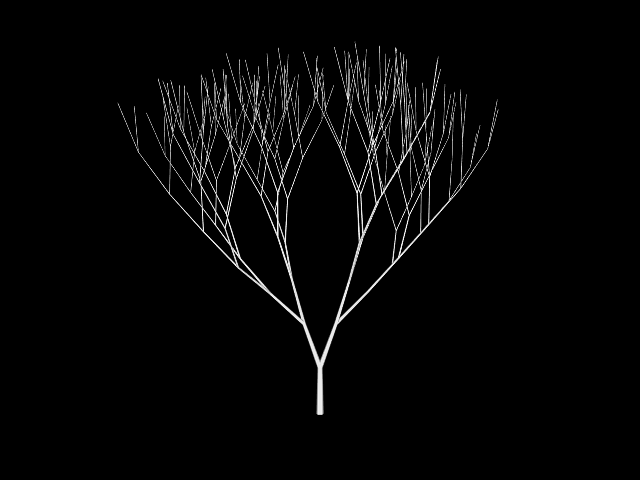```(defconstant +fibonacci+ 137.50777) (defparameter *gravity* (create-point 0.0 -1.0 0.0)) (defun segment (s) (turtle-set-surface s) (turtle-tropism 80.0 *gravity* (- (* 0.02 s) 0.7))) (with-graphics (iteration-count 14) (add-macro (R -> roll((- +fibonacci+)))) (set-axiom (R B(:active 1.0))) (add-option B (segment s)) (add-rule ((B(x s) > [ B(x1 s1) * ] [ B(x2 s2) * ] ? (<= 7 *iterations-so-far*) -> B(x (+ s1 s2))) (B(x s) ? (<= 7 *iterations-so-far*) -> B(x s)) (B(x s) ? (eq x :active) -> B(:inactive s) roll(+fibonacci+) [ +(20) B(:active 1.0) ] [ -(20) B(:active 1.0) ]) (B(x s) -> B(x s))))) ```

Fourth example illustrates that branch weight can be used to alter tropism strength. "Light" branches have negative tropism strength as if striving toward sun, while "heavy" branches have positive tropism strength as if being bent down by gravity due to their weigth.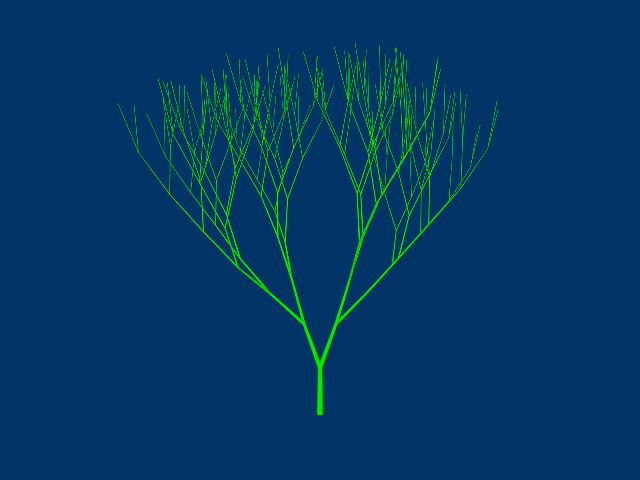```(defconstant +fibonacci+ 137.50777) (defparameter *gravity* (create-point 0.0 -1.0 0.0)) (defparameter *green* (create-color 0.0 1.0 0.0)) (defun segment (s) (turtle-set-surface s) (turtle-tropism 80.0 *gravity* (- (* 0.02 s) 0.7))) (with-graphics (iteration-count 14) (add-macro (R -> roll((- +fibonacci+)))) (set-background-color (create-color 0.0 0.2 0.4)) (set-axiom (color(*green*) R B(:active 1.0))) (add-option B (segment s)) (add-rule ((B(x s) > [ B(x1 s1) * ] [ B(x2 s2) * ] ? (<= 7 *iterations-so-far*) -> B(x (+ s1 s2))) (B(x s) ? (<= 7 *iterations-so-far*) -> B(x s)) (B(x s) ? (eq x :active) -> B(:inactive s) roll(+fibonacci+) [ +(20) B(:active 1.0) ] [ -(20) B(:active 1.0) ]) (B(x s) -> B(x s))))) ```

This and next example just add some decorations familiar from previous chapters.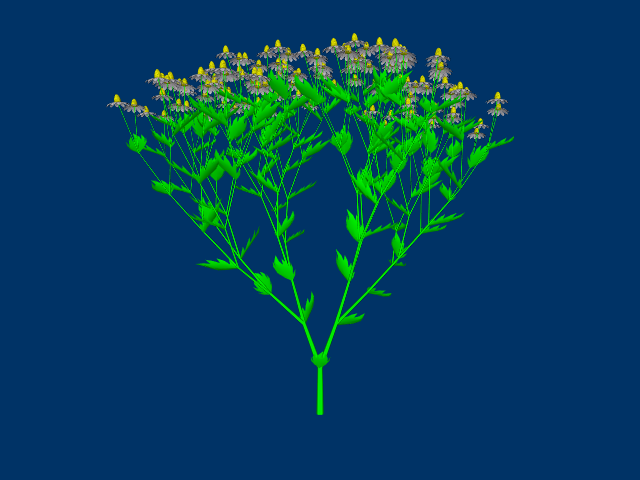```(defconstant +fibonacci+ 137.50777) (defparameter *gravity* (create-point 0.0 -1.0 0.0)) (defparameter *green* (create-color 0.0 1.0 0.0)) (defun segment (s) (turtle-set-surface s) (turtle-tropism 80.0 *gravity* (- (* 0.02 s) 0.7)) (when (= s 1.0) (turtle-object "blossom" 5.0))) (with-graphics (iteration-count 14) (add-macro (R -> roll((- +fibonacci+)))) (set-background-color (create-color 0.0 0.2 0.4)) (set-axiom (color(*green*) R B(:active 1.0))) (read-wavefront "blossom.obj" "blossom.png") (read-wavefront "leaf.obj") (add-option B (segment s)) (add-macro (L -> obj("leaf" 10.0 *green*))) (add-rule ((B(x s) > [ B(x1 s1) * ] [ B(x2 s2) * ] ? (<= 7 *iterations-so-far*) -> B(x (+ s1 s2))) (B(x s) ? (<= 7 *iterations-so-far*) -> B(x s)) (B(x s) ? (eq x :active) -> B(:inactive s) roll(+fibonacci+) [ +(20) B(:active 1.0) ] [ -(20) B(:active 1.0) ] [ roll((random 360)) +(60) L ]) (B(x s) -> B(x s))))) ```

(back to contents)